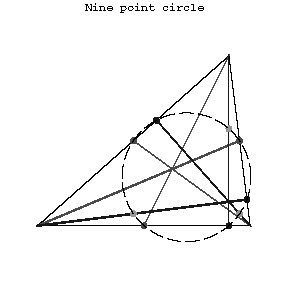# Nine-point circle

A circle whose periphery contains the midpoints of the sides of a triangle, the bases of its altitudes, and the midpoints of the segment connecting the orthocentre of the triangle with the vertices. Its radius is equal to one-half of the radius of the circle circumscribed about the triangle. The nine-point circle of a triangle is tangent to the circle inscribed in it and to the three escribed circles. Let $H$ be the orthocentre of a non-equilateral triangle, let $T$ be the centre of gravity, let $O$ be the centre of the circumscribed circle and let $E$ be the centre of the nine-point circle. The points $H,T,O,E$ then lie on a straight line (the Euler line), $E$ being the midpoint of the segment $HO$, and the pair of points $H,T$ harmonically subdivides the pair of points $O,E$.